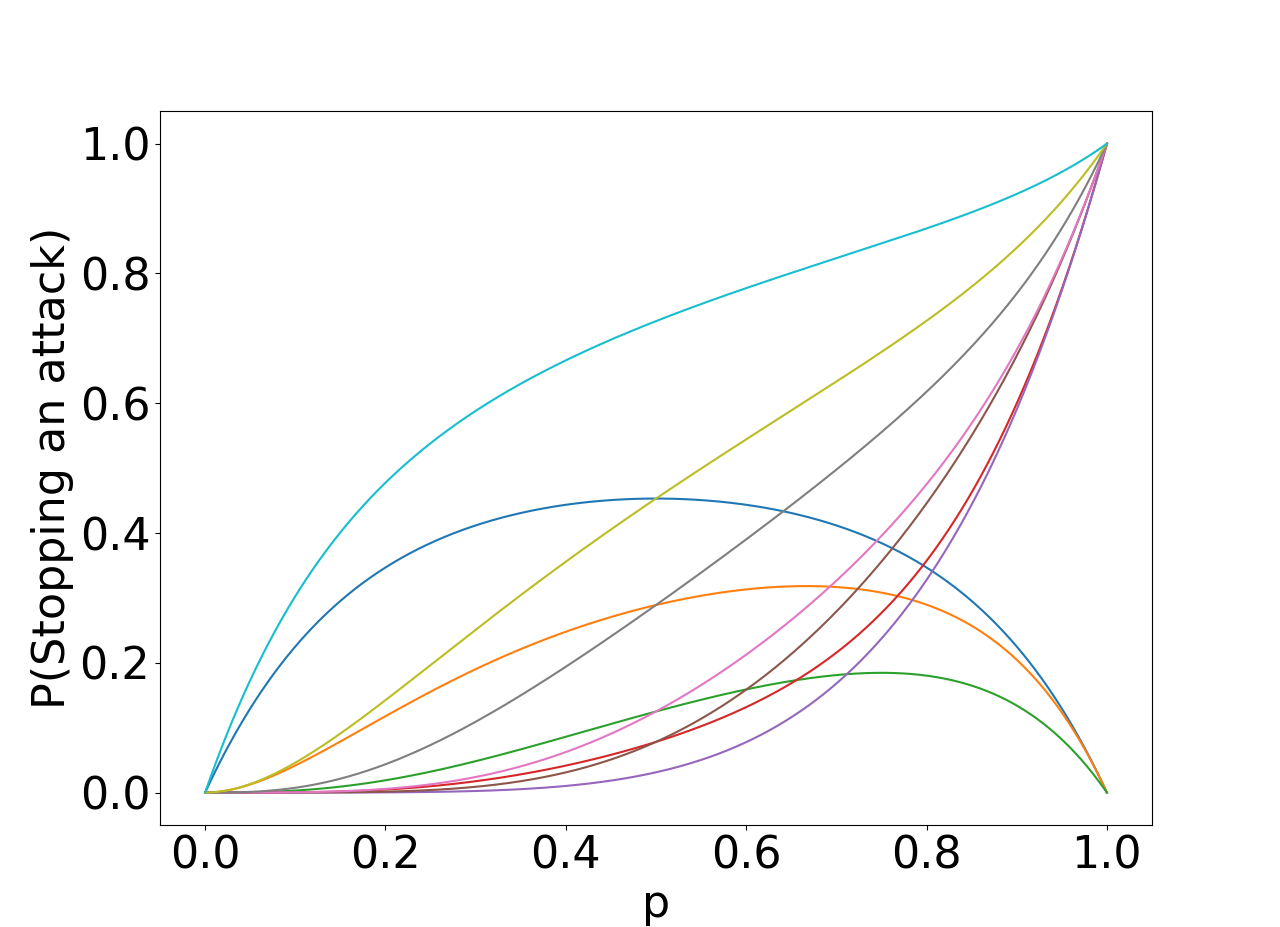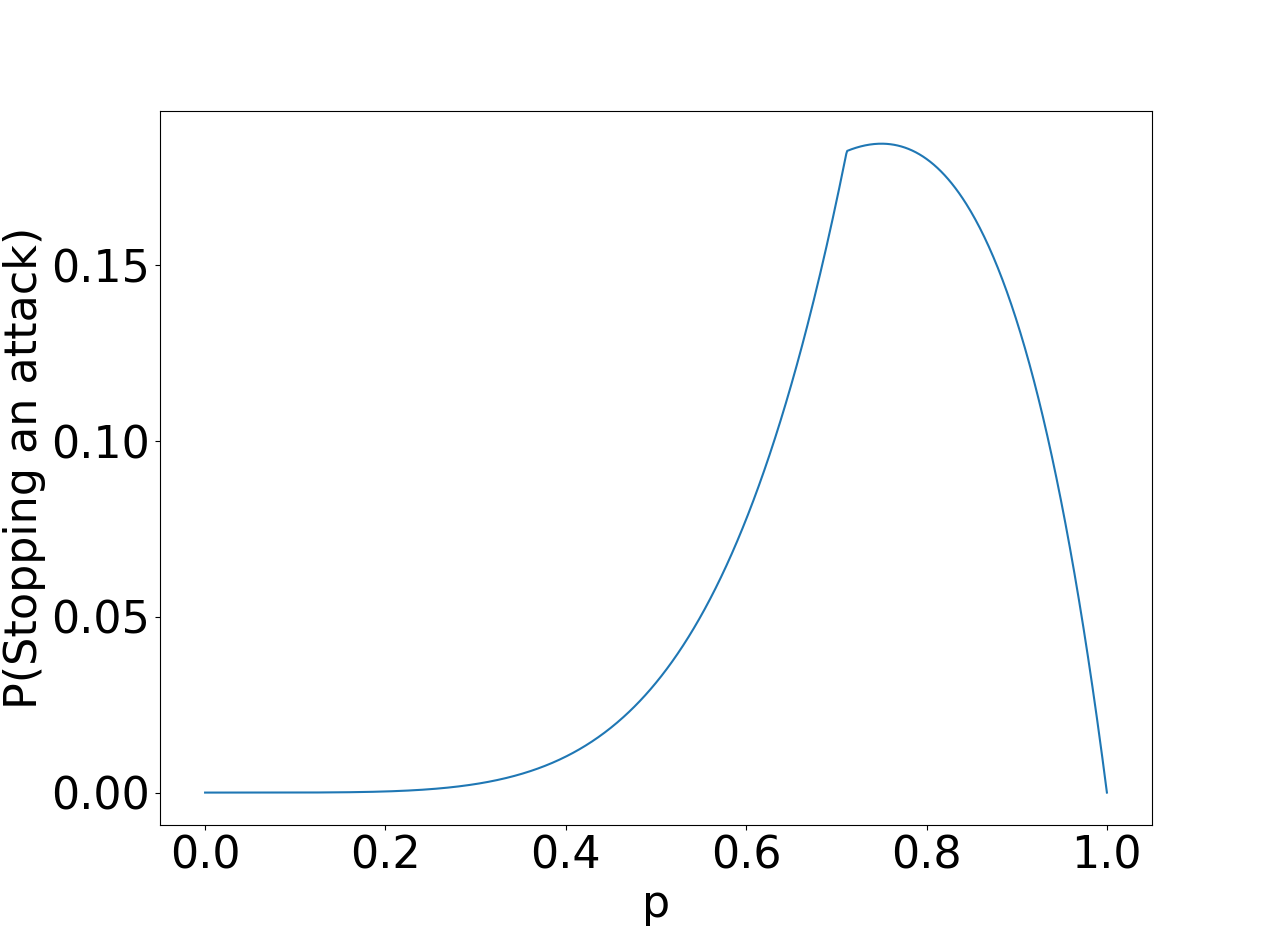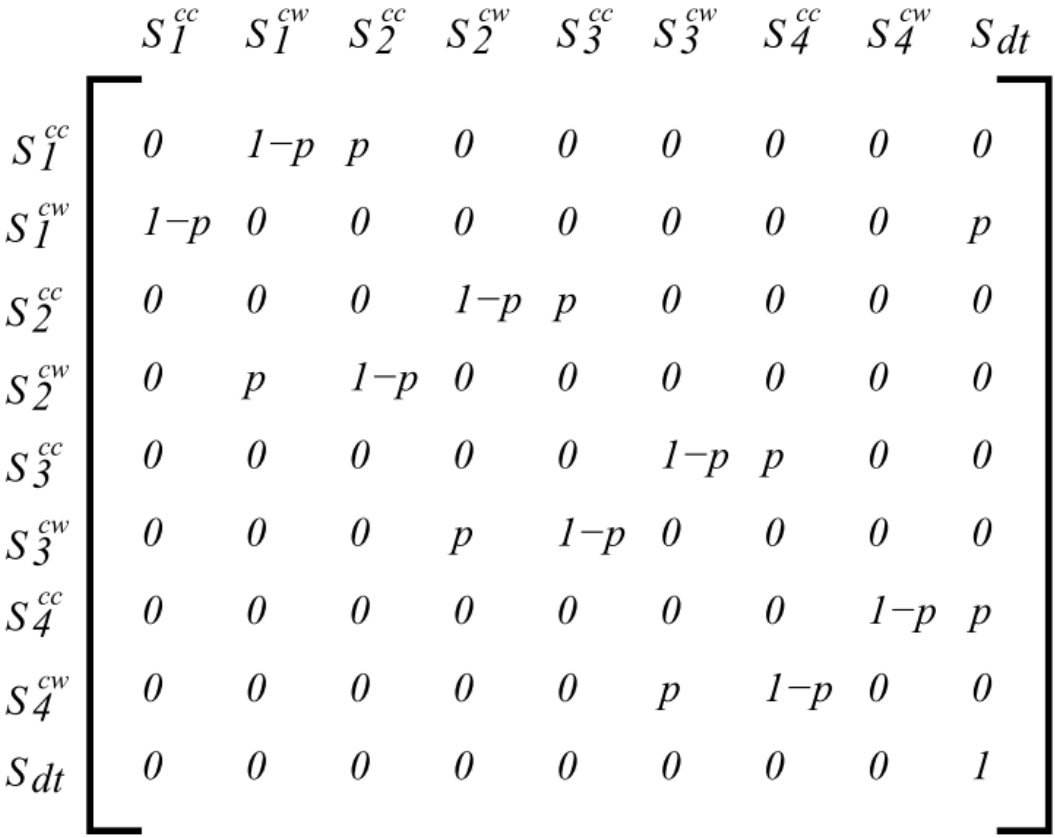Warning
This page is located in archive.

# P4 - Patrolling in polygonal environment

The main task is to implement ROS modules to gather data required to compute the optimal patrol strategy and then deploy the strategy in the environment.

 Deadline January 5th, 23:59 PST Points 10 Label in BRUTE P4-gt Evaluation upload strategy computation in strategy.py to brute and show the rest to the instructor Resources P4-gt resource package

#### Assignment

##### Introduction

The environment comprises a closed polygonal environment $P$ which is threatened by an adversary attack. To prevent this attack, the polygon $P$ is being patrolled by $k$ homogeneous mobile robots. The adversary needs to continuously attack a spot on the polygon for the duration $t$ to penetrate the polygon. The aim of this assignment is to compute and execute such a patrolling strategy that minimizes the probability of a successful attack. Such a strategy comprises dividing the polygon into segments, and changing the direction of the robot patrol.

##### Polygon segmentation

The path around the polygon $P$ may be divided into $N$ segments, where a robot traverses each segment with uniform duration. Therefore, there exists such a time-periodic function that each robot traverses exactly one segment per its period. Moreover, the robot turning action has a non-zero duration of $\tau$. It is recommended to use the turning time $\tau$ as the time unit, i.e., $\tau = 1$.Figure 1: Polygon environment and the division to segments. Note that on the polygon segments are homogeneously spaced since the segmentation is based on a uniform division of time and some actions, e.g., driving along curves, may take an increased amount of time.

First, deploy the robot along the polygon, and compute duration both the duration of circling the polygon and the duration of turning the robot, i.e., the duration to change the clockwise to counter-clockwise motion around the polygon or vice verse. Then, the trajectory may be divided into $N$ segments so that each segment is traversed by the robot in time $\tau$. It it recommended to explore different robot motion and turning velocities, since this can make the division and subsequent strategy computation easier.

##### The optimal strategy

The authors of  show that in an optimal strategy the robots are always uniformly distributed along the fence, and that at any time all the robots are always moving in a same direction. Let $d = \frac{N}{k}$ be the distance in segments between neighbor robots. Then if $t \geq d$ the strategy is trivial since it is sufficient for the robots to move forward in an arbitrary direction as such a behavior will always intercept any attacker. Conversely, if $t < \frac{d+\tau -1}{2}$, $\tau = 1$, and $t < \frac{d}{2}$ the attacker can always selected a point on the polygon where the penetration succeeds. Assuming that neither of the trivial cases holds, the optimal strategy is a stochastic one that may be generalized as follows: for each segment, continue moving forward with the probability $p$, or turn all robots at once with the probability $1-p$.

To compute the optimal $p$, consider the segments between two neighbor robots. Note, due to the symmetric nature of the problem, this applies for all such segments. A Markov chain may be used to model the possible states and transitions between states in such system. For each segment $s_i$ in the original path, consider two states in the graphic model G; one state for being on $s_i$ and moving clockwise ($s_i^{cw}$), and one for moving counterclockwise ($s_i^{cc}$). Since $\tau = 1$, the edges of G are defined as follows. The robot direction change is represented by an $1-p$ probability edge, which leads from $s_i^{cc}$ to $s_i^{cw}$. Similarly, an $1-p$ probability edge leads from $s_i^{cw}$ to $s_i^{cc}$. Finally, edges with probability $p$ lead from $s_i^{cw}$ to $s_{i-1}^{cw}$, and from $s_i^{cc}$ to $s_{i+1}^{cc}$, respectively. Note, if the index of the target state of an edge would be lower than one or bigger than $d$, the edge goes instead to the absorbing state $s_{dt}$.

The probability $p$ may be computed as follows

Create a matrix of size [2d + 1, 2d + 1], initialized with 0s
Fill out all entries in M as follows:
M[2d, 2d] = 1
for i = 0, i < 2d, i++ do
M[i,(2(i mod 2==0 ? 1 : -1)+i) mod (2d+1)] = p
M[i,((i mod 2==0 ? 1 : -1)+i) mod (2d+1)] = 1-p
end
MT = M^t
Res = vector of size d initialized with 0s
for loc = 0, loc ≤ 2d, loc++ do
V = vector of size 2d+1 initialized with 0s
V[2loc]=1
Res[loc] = V × MT[2d]
end
return Res


The algorithm returns a list of values, one for each of the $d$ segments. The value denotes the probability of stopping an attack in the segment given $p$. Therefore, to minimize the probability of a successful attack, optimize over values of $p$ from 0 to 1. Note, thousand or even hundred values should be sufficient. For each value of $p$ compute the worst-case segment, i.e., the segment with the lowers probability of stopping the attack. Then, choose $p$ that maximizes this worst-case.a) All segment probabilities for all $p$s; b) the worst cases. The selected maximum over the worst cases corresponds to $p = 0.75$; c) matrix M after first iteration.

To solve the problem, implement the following two functions, generate_function and optimal_strategy and upload them to BRUTE in file strategy.py. The generate_function function is prescribed as follows:

def generate_function(d, t, p):
"""
Function to generate the probability of stopping an attack for each segment given some p

Parameters
----------
d:int
number of spaces between two closest agents
t:int
the time required by the attacker to breach the fence
p:float
probability of continuing forward

Returns
-------
f:float[d]
list of probabilities of stopping an attack for each segment
example behavior: input: $(3,2,0.5)$, returns: $[0.25,0.25,0.5]$.

The optimal_strategy function has the following prescription

def optimal_strategy(d, t, splits):
"""
Function to generate the probability of stopping an attack for each segment given some p

Parameters
----------
d:int
number of spaces between two closest agents
t:int
the time required by the attacker to breach the fence
splits:int
a number of values of p from 0 to 1 to be created, e.g. for splits = 3, we will have p in [0,0.5,1]

Returns
-------
f_all:float[d][splits]
list of lists, one for each segment, and in the list for the segment are probabilities of stopping an attack for all possible p
f_min:float[splits]
list of minimal probabilities of stopping an attack for each p
strategy:float
value of the optimal p
prob:float
the worst-case probability of stopping an attack given strategy

example behavior: input: $(3,2,3)$, returns: $([[0.0, 0.25, 0.0], [0.0, 0.25, 1.0], [0.0, 0.5, 1.0]], [0.0, 0.25, 0.0], 0.5, 0.25)$

#### Deployment

The task is to deploy multiple robots. First, deploy a robot along the polygon and compute duration both the duration of circling the polygon and the duration of turning the robot, i.e., the duration to change the clockwise to counter-clockwise motion around the polygon or vice versa. Second, divide the polygon into segments and compute the optimal strategy $p$. Finally, space the robots equally along the polygon and deploy them while changing direction the patrol with probability $1-p$ for each segment. The direction change check may called, e.g., by ticks with $\tau$ period, or every time a lead robot enters a new segment. After a while, an attacker chooses a point on the polygon to penetrate. If one of the robots moves close enough to the point of attack, the attack is successfully prevented.

Note that for some settings, even with optimal p, the expected ratio of prevention can be as bad as 0.2.

##### Simulator

The simulator provides and processes the following inputs and outputs, respectively

 Message type ROS Topic Description Outputs Robot pose (nav_msgs/Odometry) /robot{i}/odom Robot pose provided by a simulator Polygon (geometry_msgs/PolygonStamped ) /polygon The polygon to be patrolled Inputs Velocity command (geometry_msgs/Twist ) /robot{i}/cmd_vel Velocities to drive the robot

subject to $$i \in {0,1,\ldots,k-1}$$ where k is number of robots in the simulation. Thus, the velocity command topic for the first robot is /robot0/cmd_vel.

To run the STDR simulator

1. launch the ROS node

roslaunch uir_tools_gt uir_gt_backend.launch [robot_n:=number_of_robots]
where the optional argument robot_n specifies the number of robots in the simulation.

#### Evaluation

The strategy computing functions should submitted in Brute. A demonstration in STDR is evaluated by an instructor during the labs.

#### Suggested architecture

##### Path following module

The path following module drives a robot along a given path by publishing velocity commands. The followed path represents a tour along the polygon locations and should be followed indefinitely. Moreover, an individual instance of this node should be created for each of the robots.

 Message type Topic Inputs Path (nav_msgs/Path) /robot{i}/path Path to be followed by the robot Robot odometry (nav_msgs/Odometry) /robot{i}/odom Robot position and orientation provided by a simulator Outputs Velocity command (geometry_msgs/Twist ) /robot{i}/cmd_vel Velocities to drive the robot
##### Path planning module

The path planning module plans a closed loop path over the polygon assigned to a robot, and changes the direction of this path according to the computed strategy.

 Message type Topic Inputs Robot pose (nav_msgs/Odometry) /robot{i}/odom Robot pose provided by a simulator Polygon (geometry_msgs/PolygonStamped ) /polygon The polygon to be patrolled Outputs Path (nav_msgs/Path) /robot{i}/path Path followed by the robot

#### Reference

 Agmon, Noa, Gal A. Kaminka, and Sarit Kraus. “Multi-robot adversarial patrolling: facing a full-knowledge opponent.” Journal of Artificial Intelligence Research 42 (2011): 887-916.

#### Appendix

##### Setup and ROS Basics

To run the ROS nodes, it is strongly recommended to use either Ubuntu 16 with ROS Kinetic or Ubuntu 18 with ROS Melodic. Detailed installation instructions may be found on ROS web pages ROS Kinetic, ROS Melodic.

On the lab computers, ROS Kinetic is already preinstalled but two more libraries need to be installed before using it.

pip install rospkg
pip install netifaces
Moreover, it is necessary to source the main ROS setup script, which has to be done in each new terminal instance. Thus, it is recommended to add the following source command in your ~/.bashrc file
echo "source /opt/ros/kinetic/setup.bash" >> ~/.bashrc
or alternatively when running ROS Meloding
echo "source /opt/ros/melodic/setup.bash" >> ~/.bashrc

Finally, on Ubuntu 16 the STDR simulator is installed using

sudo apt-get install ros-kinetic-stdr-*
On Ubuntu 18, STDR has to be compiled from source, which can be found at https://github.com/stdr-simulator-ros-pkg/stdr_simulator.

##### ROS Basics

On Ubuntu 16 and 18 the ROS workspace is created as follows

cd ~/
mkdir rds_ws
mkdir rds_ws/src
cd rds_ws
catkin_make

# source the workspace
cd devel
devel_path=$(pwd) echo "source$devel_path/setup.bash" >> ~/.bashrc
source setup.bash

# Add new (custom) package to the workspace
cd ~/rds_ws/src                 # Go to src folder in your workspace.
ln -s [path to your package]    # Create symlink to your package (or place the whole package directly to src).
cd ~/rds_ws
catkin_make                     # Compile the workspace.

rospack list                    # Make sure that your pack is known. (Sometimes this is necessary to refresh ROS. )
# Now, you should be able to use the package within ROS (rosrun or roslaunch).

# useful commands
rostopic list              # all current topics in the ROS system
rostopic info [topic name] # show info about topic - publishers, subscribers
rostopic echo [topic name] # writes msgs data on certain topic to stdout
rostopic hz [topic name]   # prints frequency of msgs on a certain topic

rosnode list               # all currently running nodes
rosnode info [node name]   # info about the node: published topics, subscribed topics

# useful tools
rqt_graph                  # visualize connection between the nodes (might not be 100% accurate)
rviz                       # spatial visualization of the msgs
rosrun tf view_frames      # creates pdf with a visualization of the whole transformation tree (all the /tf msgs in the system)
rosbag record              # capture ROS msgs on selected topics (data can be played with selected speed afterwards)
rosbag play [bagfile]      # play data saved in bagfile

The main study material for ROS is http://wiki.ros.org/ROS/Tutorials. A special attention should be given to ROS message-based communication presented in http://wiki.ros.org/ROS/Tutorials/WritingPublisherSubscriber%28python%29.

courses/b4m36uir/projects/p4-gt.txt · Last modified: 2020/01/02 11:27 by milecdav